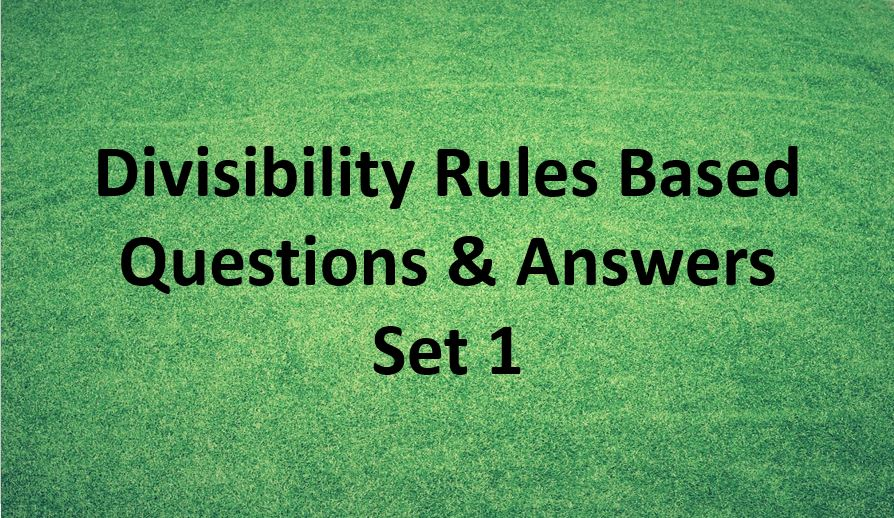Monday, September 27, 2021
Home > Latest Announcement > Divisibility Rules Question And Answer Set 1

# Divisibility Rules Question And Answer Set 1# Divisibility Rules Question and Answer Set 1

Hi students, welcome to Amans Maths Blogs (AMB). On this post, you will get the Divisibility Rules Question and Answer Set 1. It will help you to practice the questions on the topics of maths as divisibility rules of number system.

Divisibility Rules Question and Answer: Ques No 1

What is the smallest digit that should be replaced by ‘*’ in the number 296*12,  to make it divisible by 12?

Options:

A. 1

B. 2

C. 3

D. 4

Divisibility Rules Question and Answer: Ques No 2

What is the smallest positive 2-digit whole number divisible by 3 and such that the sum of its digits is 9?

Options:

A. 27

B. 33

C. 72

D. 18

Divisibility Rules Question and Answer: Ques No 3

If 8A5146B is divisible by 88, then the value of AxB is

Options:

A. 8

B. 12

C. 16

D. 14

Divisibility Rules Question and Answer: Ques No 4

What is the value of M and N respectively? If M39048458N is divisible by 8 and 11; where M and N are single digit integers

Options:

A. 7, 8

B. 8, 6

C. 6, 4

D. 5, 4

Divisibility Rules Question and Answer: Ques No 5

If M183 is divisible by 11, find the value of the smallest natural number M ?

Options:

A. 5

B. 6

C. 7

D. 8